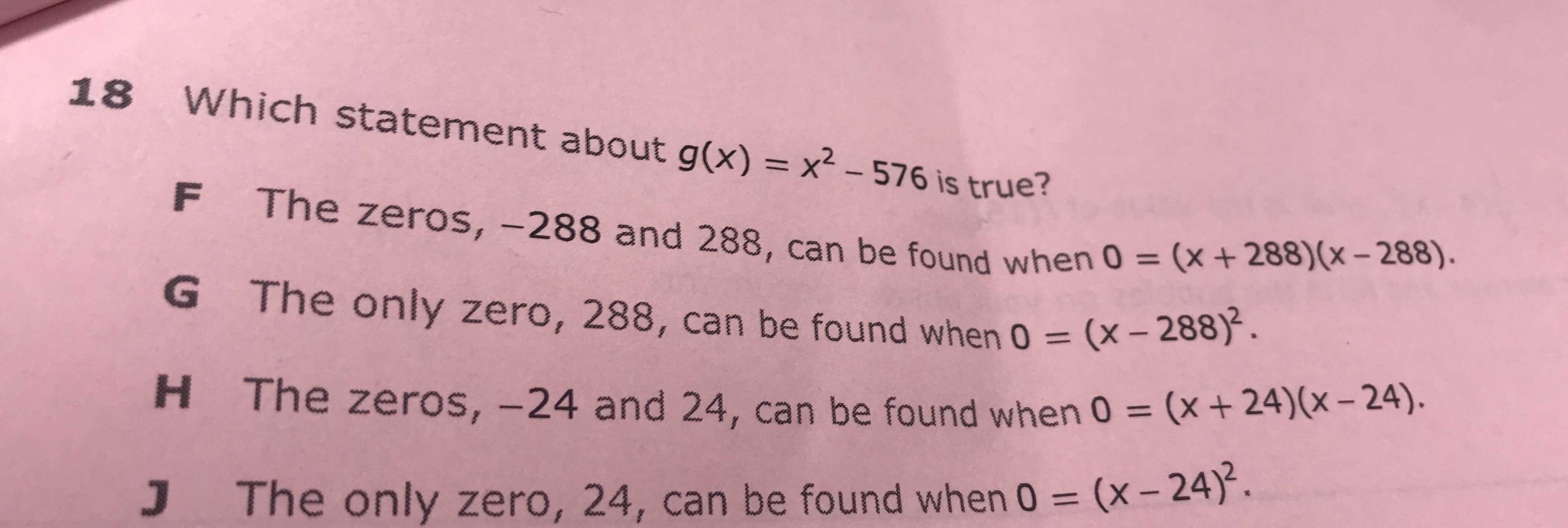### ¿Todavía tienes preguntas de matemáticas?

Pregunte a nuestros tutores expertos
Algebra
Pregunta$$18$$ Which statement about $$g ( x ) = x ^ { 2 } - 576$$ is true?

F The zeros, $$- 288$$ and $$288$$ , can be found when $$0 = ( x + 288 ) ( x - 288 )$$ .

G The only zero, $$288$$ , can be found when $$0 = ( x - 288 ) ^ { 2 }$$ .

H The zeros, $$- 24$$ and $$24$$ , can be found when $$0 = ( x + 24 ) ( x - 24 )$$

J. The only zero, $$24$$ , can be found when $$0 = ( x - 24 ) ^ { 2 }$$ .